# Fluid Theory

Plasma fluid equations are obtained by taking low-order velocity-space moments of the kinetic equation .

The low-order moments of the distribution function,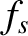, all have simple physical interpretations. First, we have the particle number density,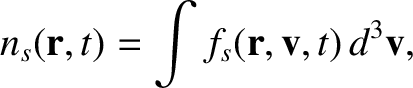(2.2)

and the mean flow velocity,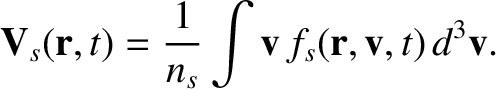(2.3)

Next, we have the pressure tensor,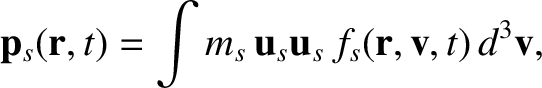(2.4)

and the heat flux,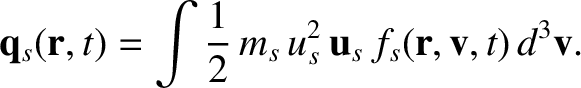(2.5)

Here,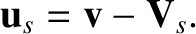(2.6)

The trace of the pressure tensor measures the ordinary (or scalar) pressure,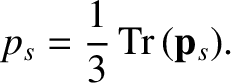(2.7)

The (kinetic) temperature is defined as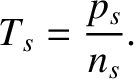(2.8)

The low-order velocity-space moments of the collision operator also have simple interpretations. The friction force density takes the form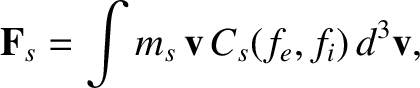(2.9)

whereas the collisional heating rate density (in the species-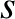rest frame) is written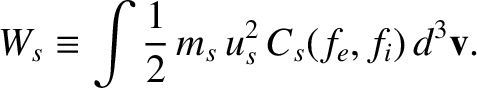(2.10)

The zeroth, first, and contracted second velocity-space moments of the kinetic equation, (2.1), yield the following set of fluid equations for species-: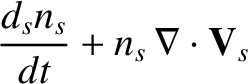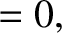(2.11)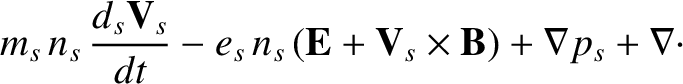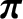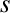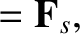(2.12)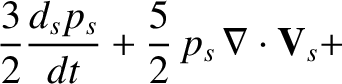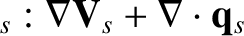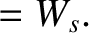(2.13)

Here,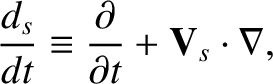(2.14)

is the well-known convective derivative, and we have written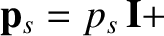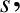(2.15)

where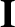is the unit (identity) tensor, and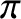is the viscosity tensor. Obviously, Equation (2.11) is a particle conservation equation for species-, Equation (2.12) is a momentum conservation equation, and Equation (2.13) is an energy conservation equation .